# Solution assignment 03 Linear functions and graphs

### Assignment 3

Draw the line: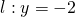and calculate the slope and the exact intersection point of the line with the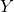-axis.

### Solution

In this case the slope of the line equals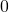(, the coefficient of, is). The line is parallel to the-axis for the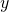-coordinate is equal to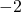for all values of. The intersection point with the-axis is.